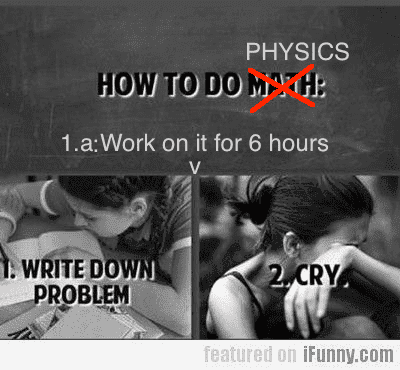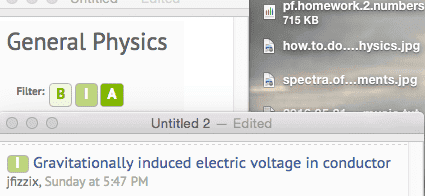# Gravitationally induced electric voltage in conductor

Gold Member
Here's a random thought that I don't as yet have a satisfactory solution:

Let's assume we have a copper column resting on an insulated table.

Copper is an electric conductor, which means that some electrons within the copper column move freely throughout the column, subject to electromagnetic repulsion between other electrons, gravity, and being confined to be within the block.

If we assume a square column that's 10 cm to a side, and 1 m in height, what would be a good estimate (order of magnitude) for the voltage difference between the top and bottom of the column?

The atoms of copper have more or less fixed locations, while the conduction electrons can flow freely. Because of gravity, I would assume that the electron density would be larger at the bottom of the column than on top, and that this charge imbalance would amount to a voltage difference. This voltage must be pretty small, otherwise the peaks of metal towers would have a significant positive charge which would drastically affect the air around them not unlike an ionic breeze machine.

Any ideas?

marcusl
Gold Member
You should be able to figure it out by balancing the gravitational potential against the Coulomb potential--attraction caused by charge separation that leaves positively charged crystal ions at top and Coulomb repulsion due to excess negative charges at bottom. Given that the ratio of gravitational to electric force is absolutely astronomical, I'm sure you can't see any effects.

phinds
Gold Member
2021 Award
You should be able to figure it out by balancing the gravitational potential against the Coulomb potential--attraction caused by charge separation that leaves positively charged crystal ions at top and Coulomb repulsion due to excess negative charges at bottom. Given that the ratio of gravitational to electric force is absolutely astronomical, I'm sure you can't see any effects.
Yeah, what Marcus said.

Dale
Mentor
2021 Award
I would think that thermal effects would have to factor in too. Not sure how.

phinds
Gold Member
2021 Award
I would think that thermal effects would have to factor in too. Not sure how.
Dale, that comment puzzles me. At 1m high, I wouldn't expect a significant temperature differential top to bottom (of course if there is, it would likely cause a greater effect than any gravitational effects).

Dale
Mentor
2021 Award
I was thinking along the lines that if the non thermal effects cause separation at some given energy, but the thermal energy may be so much greater than that separation energy so as to completely swamp any signal.

Last edited:
marcusl
Gold Member
The crystal lattice will also compress elastically under its own weight, so any effect is the net imbalance between the two.

It should be easy to work out the voltage from the gravitational potential energy. The energy for each meter is g * electron mass = 8.8*10^(-30) joule per meter for each electron. so that's 5.5 * 10^(-11) eV/me for an electron. An electric field of 5.5*10^(-11) V/m will cancel gravity. I don't think that's large enough to measure, because contact potentials of the materials involved will be much bigger.
You can't measure This voltage with an ordinary voltmeter anyway, because gravity will also work on the electrons in the leads and in the voltmeter, and this will cancel, because the gravitational potential around a closed loop is zero

OmCheeto
Gold Member
Good grief! What a great question.
After working on this for 6 hours, I've decided I have too many holes in my knowledge to even touch this.
But I did learn a lot.... while the conduction electrons can flow freely. ...
Any ideas?

I reduced the problem to a single strand of copper atoms, 1 meter high.
There are approximately 3,543,827,027 atoms in such a vertical column, weighing in at 3.23E-21 kg.
This gives a columnar force on the bottom electron of 3.17E-20 newtons.
The repulsive force on the valence electrons between each atom is about 2.89E-09 newtons.

The reason I chose to quote your "flow freely" comment, is because I found out that the ionization energy for copper is 7.73 ev/atom.

For some reason, I can not figure out how to determine if the 2.89e-9 newtons is enough to generate 7.73 electron volts.
As when I checked out Electric Potential Energy
they said that U=kQq/r
which did not seem to match the F=k|Qq|/r^2 I had seen earlier.•Drakkith
marcusl
Gold Member
Ionization energy is irrelevant here because electrons in the conduction band of the crystal form an "electron gas" that conducts freely. As for the two formulas you quote, one is for potential (energy), the other is force, so they are consistent.

BTW, I think willem2 has the right idea.

OmCheeto
Gold Member
...electrons in the conduction band of the crystal form an "electron gas" that conducts freely.
Sounds like superconductivity to me.As for the two formulas you quote, one is for potential (energy), the other is force, so they are consistent.
oh...... Um..... It was either late, or early.BTW, I think willem2 has the right idea.

I have no idea what willem2 was describing.

marcusl
Gold Member
Sounds like superconductivity to me.
No, not even close. Take a look at conduction in metals, or at free electron models
https://en.wikipedia.org/wiki/Free_electron_model
I have no idea what willem2 was describing.
He is balancing the gravitational potential energy gained by moving downwards against the electrostatic energy potential that is developed due to charge separation (electrons moving down expose positively charged ions in the copper crystal, at top). These will be equal in equilibrium.

Drakkith
Staff Emeritus

Don't look at me. This is a bit beyond my skill level.

phinds
Gold Member
2021 Award
Don't look at me. This is a bit beyond my skill level.
Hey, so is everything else. Just do what you always do and make stuff up•Drakkith
OmCheeto
Gold Member
No, not even close. Take a look at conduction in metals, or at free electron models
https://en.wikipedia.org/wiki/Free_electron_model
The following is the extent to what I understood in the wiki article: In solid-state physics, the free electron model is a simple model...

Given that all of the sections, except for one, involve quantum mechanics, I'll just excuse myself from even thinking about solving this problem.
He is balancing the gravitational potential energy gained by moving downwards against the electrostatic energy potential that is developed due to charge separation (electrons moving down expose positively charged ions in the copper crystal, at top). These will be equal in equilibrium.

Ok then.....
Don't look at me. This is a bit beyond my skill level.

You were supposed to say; "The "I" in this case does not mean "intermediate". It means "infinitely over Om's head", and you have my permission to "Unwatch" this thread. We will not think less of you. Now go do some gardening, old man".ps. I did do some more calculations, and discovered that a one atom wide wire of copper, one meter long, has a theoretical resistance of 326 billion ohms, and the resistance between two adjacent atoms is 92 ohms. I thought that was interesting. Not sure if it means anything.

•Drakkith
marcusl
Gold Member
Who says copper is a good conductor?Gold Member
This question's definitely harder than I thought!

Originally I thought something like the barometric equation would suffice, but there's no reason to think the conduction electrons behave like an ideal gas. Also, where the electron gas is rarefied, there is a net positive charge due to the exposed copper ions, which will produce a net upward force on the electrons below, which means the barometric equation wouldn't work anyway. A proper solution probably requires knowing simplified approximations of magnetohydrodynamics, which I don't. Plasma physicists, and geophysicists may know how to do this.

The most coarse, simplified estimate I can think of would be to assume the electrons are uniformly distributed in a rigid volume equal to the volume of the block, and to let that volume overlap with the positive copper ions in the block. An electrically neutral block of copper would then be two blocks: an ion block and an electron block overlapping each other. As you move the electron-block down, there will be some distance where the upward coulomb force from the exposed ions will be enough to counteract the weight of the electron block. If we assume this model, what would the voltage difference be? As an added simplification, we can assume this displacement is small relative to the length of the block so that we're basically finding the voltage difference between the exposed positive ions on top and the exposed electrons on the bottom.

My estimate comes out to be of the order of 1000 V, which is orders of magnitude too large, and my empricial formula for the voltage is $V\approx \sqrt{\frac{M_{e}}{4\pi \epsilon_{0}}}$, where $M_{e}$ is the total mass of the free conduction electrons in the block (assuming one per atom), and $\epsilon_{0}$ is the permittivity of free space. I've almost certainly made a mistake somewhere down the line, but is it correct to assume that every atom in a conductor donates an electron to the conduction band?

256bits
Gold Member
but there's no reason to think the conduction electrons behave like an ideal gas

Nah. I think they act like a Fermi gas, with degeneracy pressure in the copper block.
If one wants to believe these guys, there is 40 G Pa of pressure in the block due to the degenerate electrons.
https://www.eng.fsu.edu/~dommelen/quantum/style_a/cboxdp.html

If the "mgh" of the electrons can add significantly to the 40 G Pa pressure, from the top to the bottom of the copper block, there could be something of an electrostatic potential produced.

( I honestly do hope I am not taking Phinds advice that he gave to Drakith. )

•OmCheeto and Drakkith
OmCheeto
Gold Member
Nah. I think they act like a Fermi gas, with degeneracy pressure in the copper block.
If one wants to believe these guys, there is 40 G Pa of pressure in the block due to the degenerate electrons.
https://www.eng.fsu.edu/~dommelen/quantum/style_a/cboxdp.html

...
Whoever wrote that article, gets my vote for "funniest" scientist of all time.
Of course, degeneracy pressure is a poorly chosen name. It is really due to the fact that the energy distribution of electrons is not degenerate, unlike that of bosons. Terms like exclusion-principle pressure or “Pauli pressure” would capture the essence of the idea. So they are not acceptable.
...
Obviously, electrons are not very smart.

I can so relate, with electrons........

•256bits and Drakkith
•